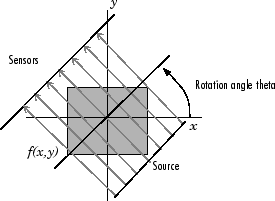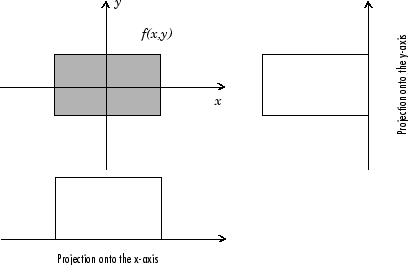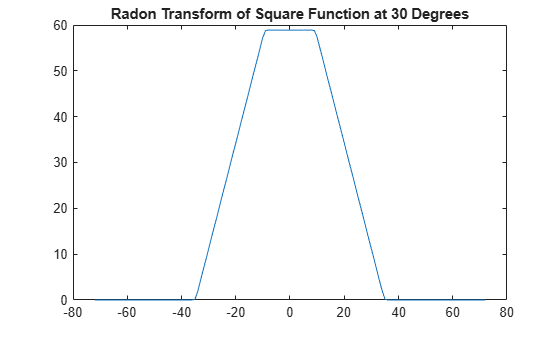Note

For information about creating projection data from line integrals along paths that radiate from a single source, called fan-beam projections, see Fan-Beam Projection. To convert parallel-beam projection data to fan-beam projection data, use the para2fan function.

The radon function computes projections of an image matrix along specified directions.

A projection of a two-dimensional function f(x,y) is a set of line integrals. The radon function computes the line integrals from multiple sources along parallel paths, or beams, in a certain direction. The beams are spaced 1 pixel unit apart. To represent an image, the radon function takes multiple, parallel-beam projections of the image from different angles by rotating the source around the center of the image. The following figure shows a single projection at a specified rotation angle.

Parallel-Beam Projection at Rotation Angle ThetaFor example, the line integral of f(x,y) in the vertical direction is the projection of f(x,y) onto the x-axis; the line integral in the horizontal direction is the projection of f(x,y) onto the y-axis. The following figure shows horizontal and vertical projections for a simple two-dimensional function.

Horizontal and Vertical Projections of a Simple FunctionProjections can be computed along any angle theta (θ). In general, the Radon transform of f(x,y) is the line integral of f parallel to the y´-axis

${R}_{\theta }\left(x\prime \right)={\int }_{-\infty }^{\infty }f\left(x\prime \mathrm{cos}\theta -y\prime \mathrm{sin}\theta ,x\prime \mathrm{sin}\theta +y\prime \mathrm{cos}\theta \right)dy\prime$

where

The following figure illustrates the geometry of the Radon transform.Plot the Radon Transform of an Image

This example shows how to compute the Radon transform of an image, I, for a specific set of angles, theta, using the radon function. The function returns, R, in which the columns contain the Radon transform for each angle in theta. The function also returns the vector, xp , which contains the corresponding coordinates along the x-axis. The center pixel of I is defined to be floor((size(I)+1)/2), which is the pixel on the x-axis corresponding to x' = 0.

Create a small sample image for this example that consists of a single square object and display it.

I = zeros(100,100);
I(25:75,25:75) = 1;
imshow(I)Calculate the Radon transform of the image for the angles 0 degrees and 45 degrees.

Plot the transform for 0 degrees.

figure
plot(xp,R(:,1));
title('Radon Transform of a Square Function at 0 degrees')Plot the transform for 45 degrees.

figure
plot(xp,R(:,2));
title('Radon Transform of a Square Function at 45 degrees')Viewing the Radon Transform as an Image

The Radon transform for a large number of angles is often displayed as an image. In this example, the Radon transform for the square image is computed at angles from 0° to 180°, in 1° increments.

theta = 0:180;